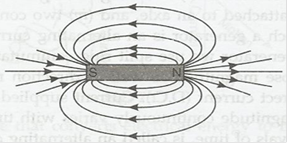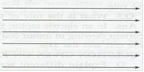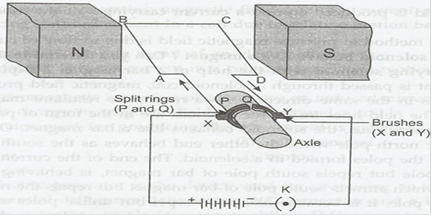### Magnetic Effects of Electric Current - Solutions

CBSE Class 10 Science

NCERT Solutions
Chapter - 13
Magnetic Effects of Electric Current

Page No. 224

1. Why does a compass needle get deflected when brought near a bar magnet?

Ans. The compass needle is small bar magnet. When a compass needle is brought near a bar magnet then due to repulsive force between unlike poles and attraction between unlike poles, the compass needle is deflected and settle in the direction of net magnetic field.

Page No. 228

1. Draw magnetic field around a bar magnet.

Ans. Magnetic field lines are as follows:2. List the properties of magnetic lines of force.

Ans. Properties of magnetic field lines of force as follows:

a. Outside a magnet, the field lines are directed from N-pole of magnet towards S-pole and inside the magnet lines are directed form S-pole to N-pole.

b. Magnetic field lines are closed curves.

c. No two magnetic field lines intersect each other’s.

d. Relative strength of magnetic field lines is given by degree of closeness of the filed lines.

3. Why don’t two magnetic lines of force intersect each other?

Ans. No, two magnetic field lines can ever intersect each other. If they do, then it would mean that at the point of intersection there are two directions of magnetic field, which is not possible.

Page No. 229

1. Consider a circular loop of wire lying in the plane of the table. Let the current pass through the loop clockwise. Apply the right-hand rule to find out the direction of the magnetic field inside and outside the loop.

Ans. As per right-hand rule, we find that inside the loop, the magnetic field lines are directed perpendicular to the plane of paper in the inward direction. Outside the loop magnetic field lines are directed out of the plane paper.

2. The magnetic field in a given region is uniform. Draw a diagram to represent it.

Ans. The uniform magnetic field is represented by parallel equispaced lines of equal length as follows:3. Choose the correct option:

The magnetic field inside a long straight solenoid-carrying current

(a) Is zero.

(b) Decrease as we move towards its end.

(c) Increase as we move towards it end.

(d) Is the same all points.

Ans. (d) is the same at all points.

Page No. 231-32

1. Which of the following property of a proton can change while it moves freely in a magnetic field?

(a) mass

(b) speed

(c) velocity

(d) momentum

Ans. (c)(d) Velocity as well as momentum will change.

2. In activity 13.7, how do we think the displacement of rod AB will be affected if (i) current is rod AB is increased, (ii) a stronger horse shoe magnet is used, and (iii) length of the rod AB is increased?

Ans. (i) On increasing the current in rod AB its displacement will increase.

(ii) If stronger horse-shoe magnet is used then the displacement of rod AB will increase.

(iii) If length of the rod is increased, force acting on it will increase and hence, displacement of the rod increases.

3. A positively-charged particle (alpha-particle) projected towards west is deflected towards north by a magnetic field. The direction of magnetic field is :

(a) towards south

(b)towards east

(c) downward

(d) upward

Ans. (d) the direction of magnetic field is vertically upward.

Page No. 233

1. State Fleming’s left-hand rule.

Ans. Fleming’s left hand rule states that stretch the forefinger, the central finger and the thumb of your left hand mutually perpendicular to each other. If the forefinger shows the direction of the magnetic field and central finger that of the current, then the thumb will point towards the direction of motion of the conductor.

2. What is the principle of an electric motor?

Ans. An electric motor is based on the principle that the current carrying conductor experiences a force when placed in a magnetic field. If the direction of the magnetic field and that of the current are mutually perpendicular then the direction of the force is given by Fleming’s left-hand rule.

3. What is the role of the split ring in an electric motor?

Ans. In electric motor, the split ring acts as commutator. Due to its action, the direction of current flowing in motor coil reverses after half turn, giving rise to a continuous rotation of the coil and the axle.

Page No. 236

1. Explain different ways to induce current in a coil.

Ans. Different ways to induce current in a coil are as follows:

(a) If a magnetic field is changed around a coil then an induced current is set up in the coil.

(b) If a coil is moved in magnetic field, then again an induced current is set up in the coil.

(c) If a coil is rotated in a uniform magnetic field.

Page No. 237

1. State the principle of an electric generator.

Ans. An electric generator is based on the principle of electromagnetic induction. When a rectangular coil is rotated in a uniform magnetic field, an induced emf is generated between the ends of the coil.

2. Name some sources of direct current.

Ans. Some sources of direct current are a cell, a battery and a D.C. generator.

3. Which sources produce alternating current?

Ans. A.C. generator and common inverter used in houses for emergency power supply produce alternating current.

4. Choose the correct option:

A rectangular coil of copper wires is rotated in a magnetic field. The direction of the induced current changes once in each

(a) two revolutions

(b) one revolution

(c) half revolution

(d) one-fourth revolution

Ans. (c) half-rotation.

Page No. 238

1. Name two safety measures commonly used in electric circuits and appliances.

Ans. Two safety measures are:

(a) use of earth wire and proper earthing.

(b) use of fuse.

2. An electric oven of 2 kW power rating is operated in a domestic electric circuit (220V) that has a current rating of 5.A. What result do you expect? Explain.

Ans. Power rating of electric oven (P) = 2 kW = 2000 W

Current drawn (I) = P/V = 2000/220 = 9.09 A.

As the current rating of domestic electric circuit is only 5A the oven draws a current of 9.09 A. Which is more than the current rating; hence the circuit will be damaged due to overheating/overloading.

3. What precaution should be taken to avoid the overloading of domestic electric circuit?

Ans. We should take following precaution to avoid the overloading of domestic electric circuit:

(a) Two separate circuits should be used, one of 5A current rating of bulbs, fans, tubes etc. and the other 15 A current rating for appliances with higher current rating such as geysers, air coolers, electric iron, electric stoves etc.

(b) Too many appliances should never be connected to a single socket.

(c) A fuse of appropriate current rating should be used with the electric circuit.

TEXTBOOK EXERCISES

1. Which one of the following correctly describes the magnetic field near a long straight wire?

(a) The field consists of straight lines perpendicular to the wire.

(b) The field consists of straight lines parallel to the wire

(c) The field consists of radial lines originating from the wire

(d) The field consists of concentric circles centered on the wire

Ans. (d) The field consists of concentric circles centred on the wire.

2. The phenomenon of electromagnetic induction is

(a) the process of charging a body.

(b) the process of generating magnetic field due to a current passing through a coil.

(c) producing induced current in a coil due to relative motion between a magnet and the coil.

(d) the process of rotating a coil at an electric motor.

Ans. (c) producing induced current in a coil due to relative motion between a magnet and the coil.

3. The device used for producing electric current is called

(a) generator

(b) galvanometer

(c) ammeter

(d) motor

Ans. (a) generator

4. The essential difference between A.C. generator and a D.C. generator is that

(a) A.C. generator has an electromagnet while a D.C. generator has permanent magnet.

(b) D.C. generator will generate a higher voltage

(c) A.C. generator will generate a higher voltage

(d) A.C. generator has slip rings while the D.C. generator has commutator.

Ans. (d) A.C. generator has slip rings while the D.C. generator has commutator.

5. At the time of short circuit, the current in the circuit:

(a) reduce substantially

(b) does not change

(c) increase heavily

(d) vary continuously

Ans. (c) increase heavily

6. State whether the following statements are true or false.

(a) An electric motor converts mechanical energy into electrical energy.

(b) An electric generator works on the principle of electromagnetic induction.

(c) The field at the centre of a long circular coil carrying current will be parallel straight line

(d) A wire with green insulation is usually the live wire of an electric supply.

Ans. (a) False

(b) True

(c) True

(d) False

7. List three methods of producing magnetic field.

Ans. Three methods of producing magnetic fields are as follows:

(a) Magnetic field can be produced by placing a permanent magnet or a horse-shoe magnet at the place, where magnetic field is required.

(b) Magnetic field is produced around a current carrying straight conductor or a current carrying coil.

(c) A very good method to produce magnetic field is due to flow of current in a solenoid.

8. How does a solenoid behave like a magnet? Can you determine the north and south poles of a current carrying solenoid with the help of a bar magnet? Explain.

Ans. When current is passed through a solenoid coil, magnetic field is produced due to presence of turns in same direction. As a result, the resultant magnetic field is very strong and uniform. Solenoid behaves like a strong bar magnet.

We can determine the poles of magnet formed by solenoid. The end of solenoid connectd with positive terminal behaves like South Pole and the end connected with negative terminal behaves as North Pole.

9. When is the force experienced by a current-carrying conductor placed in magnetic field largest?

Ans. The force experienced by a current carrying conductor placed in a magnetic field is largest when the conductor is placed with its length in a direction perpendicular to that of magnetic field.

10. Imagine that you are sitting in chamber with your back to one wall. An electron beam, moving horizontally from back wall towards the front wall, is deflected by a strong magnetic field to your right side. What is the direction of magnetic field?

Ans. An electron beam moving horizontally from back wall towards the front wall is equivalent to a current flowing in the opposite direction. The deflection of electron beam as seen by the observer is to his right side. On applying Fleming’s left-hand rule we find that the magnetic field is acting in vertically downward direction.

11. Draw a labelled diagram of an electric motor. Explain its principle and working. What is the function of split ring in an electric motor?

Ans. Electric motor labelled diagram of an electric motor is as follows:Principle: A current-carrying conductor, when placed in a magnetic field, experiences a force. If the direction of magnetic field and that of current are mutually perpendicular, then force acting on the conductor will be perpendicular to both and will be the given by Fleming’s left-hand rule. Due to this force the conductor begins to move, if it is free to rotate.

WorkingLet the current in the coil ABCD of motor enters from the source battery through the conducting brush X, flow along ABCD and finally flows back to the battery through brush Y. On applying Fleming’s left-hand rule we find that force acting on arm AB due to magnetic field pushes it downwards. But the force acting on arm CD pushes it upwards. Thus, the coil and the axle rotate anticlockwise. Due to action of split rings P and Q change their contacts with brushes. Now, P makes contact with Y and Q with X. As a result, Current begins to flow in coil along DCBA. The arms are pushed in opposite direction and coil continues to rotate in same direction.

12. Name some devices in which electric motors are used.

Ans. Electric motors are used in all devices where we want to convert electrical energy into mechanical energy. In our houses, electric motors, coolers, mixer grinders, washing machines, computers etc motor is used.